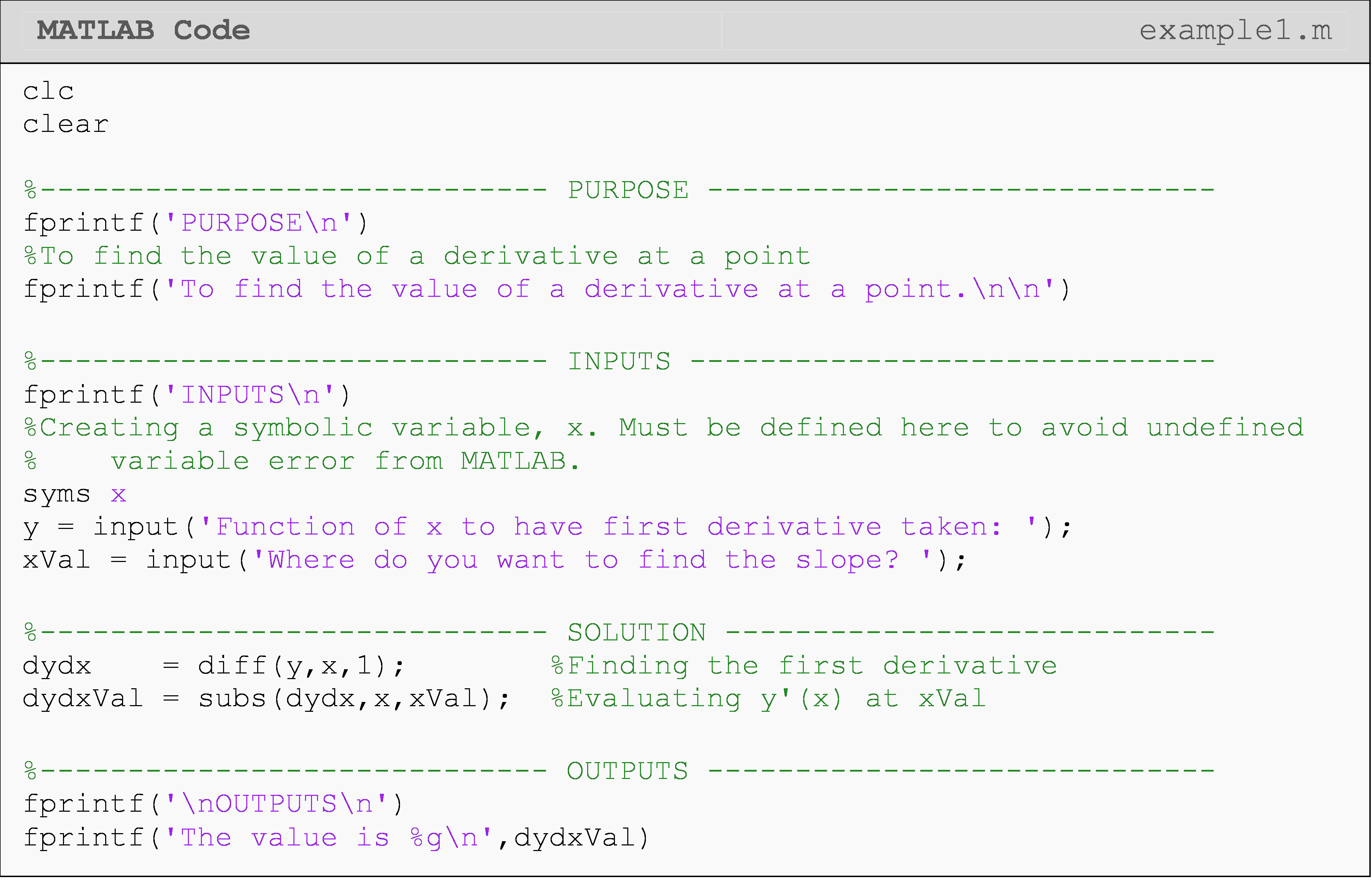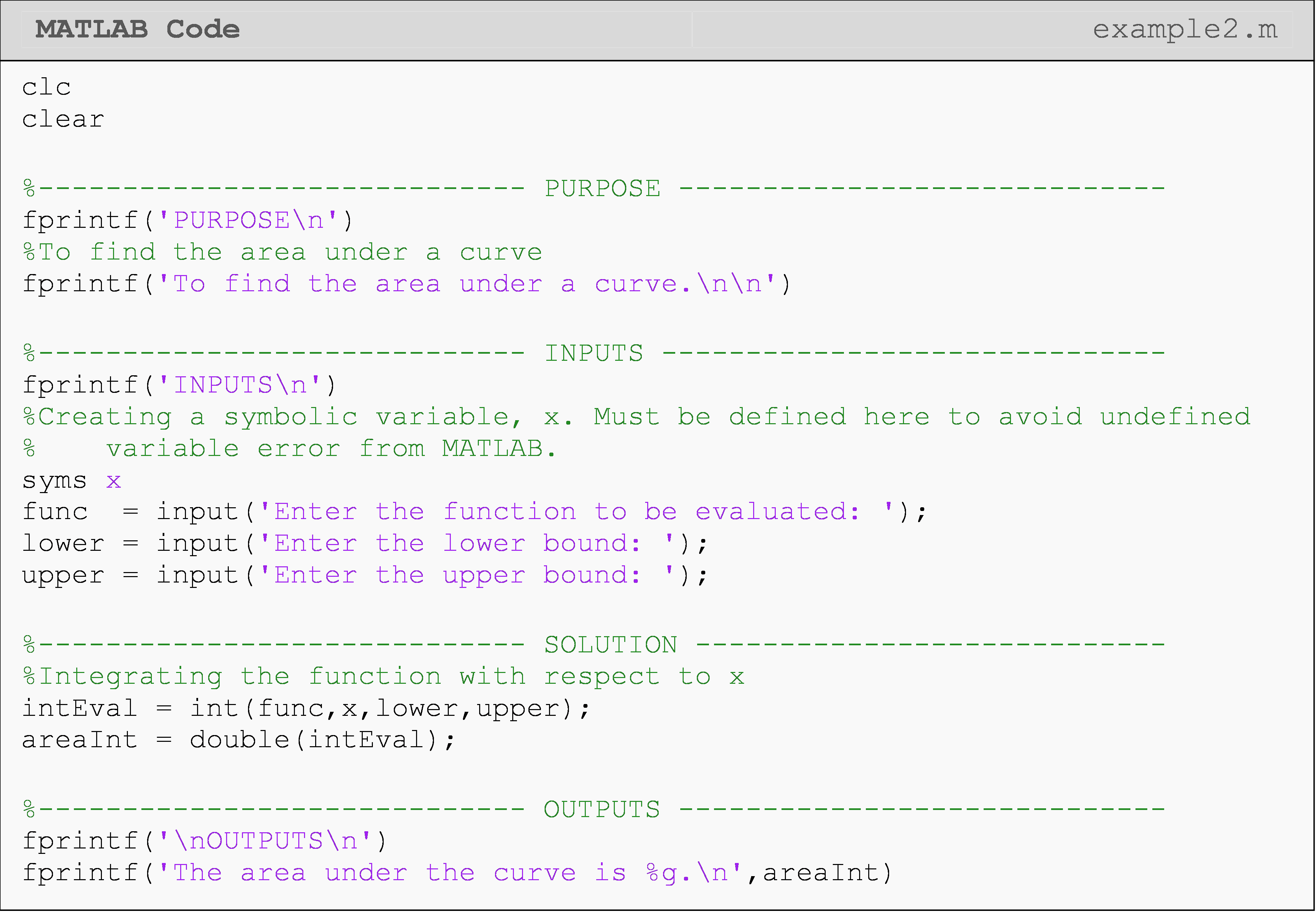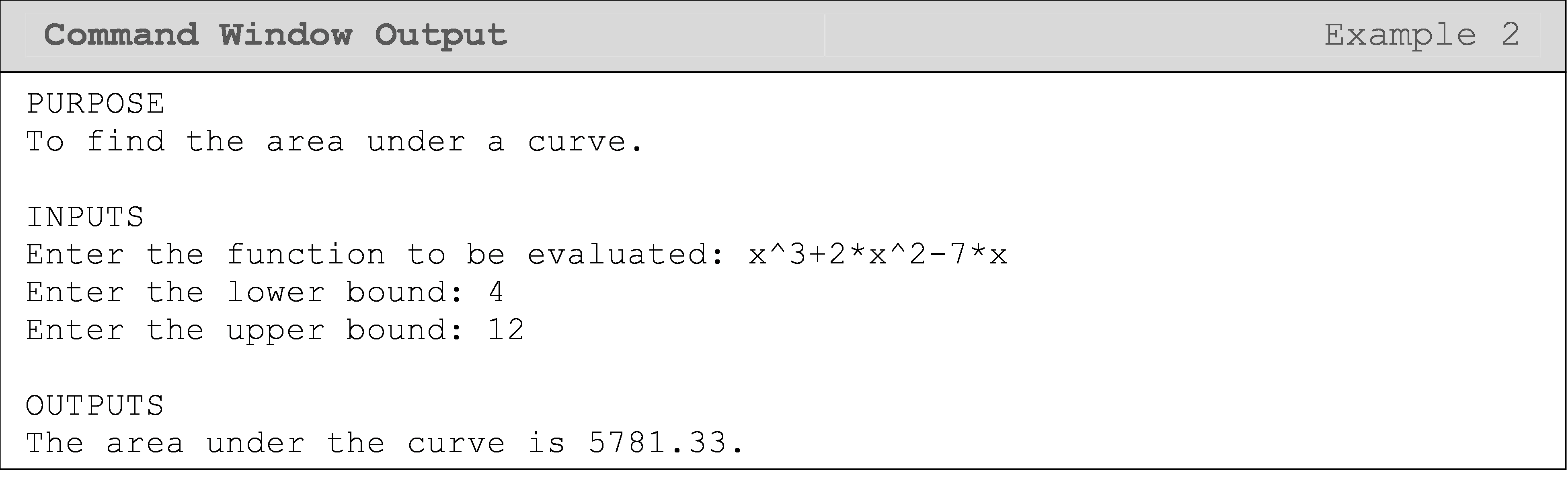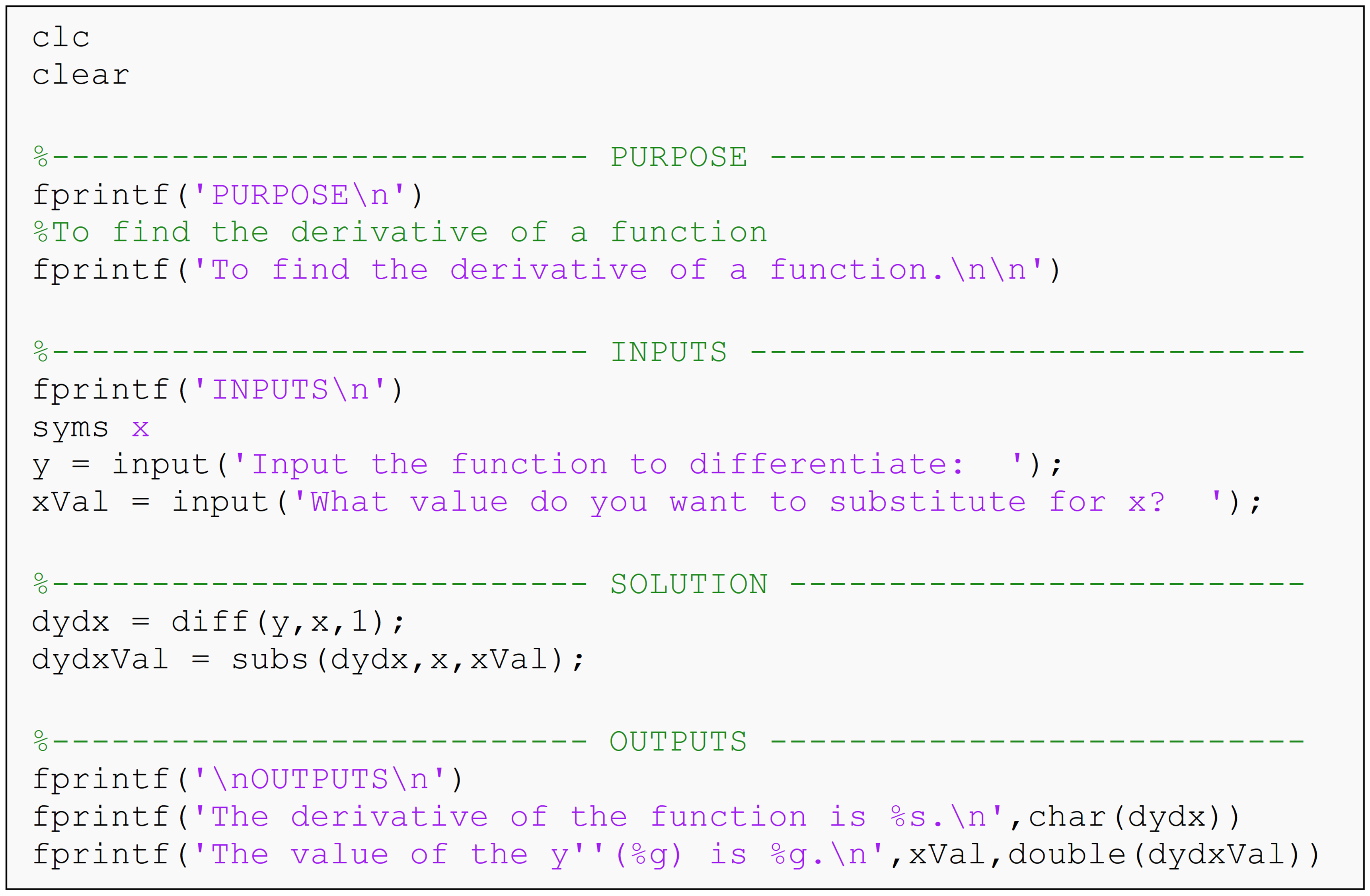# Module 6: PROGRAM DESIGN AND COMMUNICATION

## Learning Objectives

After reading this lesson, you should be able to:

• write a MATLAB program based on reading a pseudocode.

## What is a pseudocode?

A pseudocode is an informal outline of a program or computer process that is written using a mixture of computer language syntax and English conventions. When a programmer writes a pseudocode, it is intended for the use of other programmers or users, and not for a computer to read or process. Similarly, pseudocodes are used in the realm of numerical methods to communicate ideas, without having to write out long numerical equations. There are no rules for what you can or cannot state in a pseudocode, but the code needs to be easily readable, therefore subroutines and formal variable names are often omitted.

To keep the pseudocode organized, a commonly accessible word processor should be used. This makes editing, opening, and sending the pseudocode easy. Remember, the pseudocode should be easy to read and user-friendly.

When planning out code, you can also write some quick and less formal pseudocode. In this case, handwritten pseudocode is perfectly fine. As you become a more experienced programmer, these tools will become more useful and intuitive.

## How are pseudocodes used?

A good pseudocode is used to outline a specific order of events to solve a problem, and hence, bridging the gap between the problem and the solution. To solve any problem, one must first clearly identify the problem, next determine the methods to solve the problem, and finally, solve the problem. Code can generally be written faster and communicated better with the use of pseudocode(s) to design the structure of the program and outline the operations to be conducted. A pseudocode should usually be written without bias to any programming language. Keep in mind that programming languages do not always share the same syntax for the same task. For this reason, when developing a pseudocode, try to state the process desired and not the syntax needed to complete the process. Leave it to the reader to decide what syntax to use to complete the process. Example 1 shows how a pseudocode is developed.

### Example 1

Your boss has given you the MATLAB program shown in Figure 1. He tells you that the program will be converted to Python by another worker, and needs you to develop a pseudocode for the Python worker to follow. Be sure to avoid using MATLAB specific syntax in the pseudocode, as MATLAB and Python have different syntax.Solution

The pseudocode:

Find the value of the derivative of a function at a point.

1. Clear all windows and workspace variables.

2. Display the purpose of the program.

3. Define x as a symbolic variable.

4. Prompt user to enter the desired function.

Enter as a function of x.

1. Take the first derivative of input function with respect to x.

2. Prompt user to enter the point where the derivative needs to be evaluated.

3. Substitute/replace x with the number.

Substitute the value for x where derivative needs to be found.

1. Display value of the derivative of the function at a given value of x.

2. End program.

Note that the pseudocode is an informal outline containing a mixture of English and programming syntax. This makes the process easier for any reader to follow and to interpret. Even if the reader does not understand MATLAB, they should still be able to understand the process of the program. The pseudocode in Example 1 is fairly detailed and could be made more compact. However, be aware, that a fine line separates an over-detailed pseudocode from an under-detailed one, and that it is the responsibility of the writer to provide enough details to outline the process clearly.

## How can I convert a pseudocode for a problem into a program?

If you are given a problem, you can write a pseudocode by following the logic of how the problem would be solved. The pseudocode is now a roadmap to writing the MATLAB program. Fully read the pseudocode to understand the objective of the program. Once you have read the pseudocode, re-read it, this time marking the inputs and corresponding outputs. Also, you should be taking a mental (or written) inventory of the commands and functions needed to accomplish the objective. Look at Example 2 to see how a pseudocode is written for a simple problem and then turned into a program.

### Example 2

You are given the task of writing a MATLAB program for finding the value of the definite integral based on a customer’s specifications.

$\int_{a}^{b}{f\left( x \right)dx}$

The specifications state that the user is to be prompted to enter all program inputs (they are not interested in seeing the m-file) and that only the value of the integral should be displayed.

1. Write a pseudocode that outlines the problem.

2. Solve the problem using MATLAB.

Solution

Find the area under a curve.

• Clear all windows and workspace variables

• Display the purpose of the program

• Input (as prompted)

• Function, f (x)

• The lower limit of integration, a

• The upper limit of integration, b

• Integrate (f (x), x, a, b)

• Output

• The area under a curve
1. With the pseudocode provided in part (a), the following m-file has been developed. Note the order and process of the program, and compare the m-file to the pseudocode.## Multiple Choice Quiz

(1). A pseudocode is

(a)  a mixture of computer language and English.

(b)  computer language in an outline form.

(c)  English that is translated to a computer language

(d)  a waste of time.

(2). Pseudocodes are written by

(a)  humans for computers to read

(b)  humans for humans to read.

(c)  computers for computers to read.

(d)  computers for humans to read.

(3). The format for a typical pseudocode is

(a)  paragraph form.

(b)  MATLAB comment form.

(c)  a collection of abbreviations.

(d)  outline form.

(4). Computer-language specific names should be

(a)  used as little as possible in a pseudocode.

(b)  okay to use in a pseudocode.

(c)  entirely avoided in a pseudocode.

(d)  included as much as possible in a pseudocode.

(5). When writing pseudocode, the format can include _____ comments per line.

(a)  one

(b)  two

(c)  three

(d)  as many as you want

## Problem Set

(1). Write a pseudocode for a program that outputs the surface area and volume of a sphere where the input is the radius of the sphere.

(2). Write a pseudocode for a program that outputs a person’s BMI where the inputs are the person’s weight in lbs and height in inches. The BMI formula is,

$\text{BMI} = \frac{weight\ (lbs)}{\lbrack height\ (in)\rbrack^2} \times 703$

(3). Convert the MATLAB program shown below into a pseudocode.